Module 10 - Rules of Differentiation Introduction | Lesson 1 | Lesson 2 | Lesson 3 | Lesson 4 | Self-Test Lesson 10.1: The Power Rule In this lesson you will use the Derivative key on your TI-89 to develop the Power Rule for derivatives. After exploring several examples you should notice a pattern. You will then be asked to verbalize the pattern and to use it to make predictions. Once you test your predictions you will generalize the pattern to include other types of examples. Execute the NewProb command from the F6:Clean Up menu. Exploring the Derivative of xn 10.1.1 Use the Derivative key to find the derivatives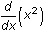,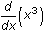, and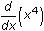. Click here for the answers. Describing the Pattern 10.1.2 Describe the pattern shown in the answers to Question 10.1.1. Click here for the answer. Predicting the Result and Testing the Prediction 10.1.3 Predict the derivatives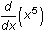and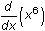then use your calculator to test your predictions. Click here for the answers. Generalizing 10.1.4 Generalize your conjecture by predicting the derivative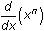and testing your prediction with your calculator. Click here for the answer. The last result,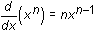, is known as the Power Rule. Extending the Power Rule You have only tried the Power Rule for exponents that are positive integers. 10.1.5 Determine if the Power Rule is valid for other types of exponents by predicting and then testing your prediction for the derivatives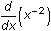,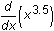, and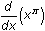. Click here for the answers. Note thatis written as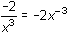, which conforms to the Power Rule. The proof of the Power Rule is not simple. You should refer to a calculus text for further details. The text will probably prove the rule first for exponents that are positive integers and then later expand the rule to other types of exponents. It can be shown that the Power Rule is valid for all real number exponents. < Back | Next > ©Copyright 2007 All rights reserved. | Trademarks | Privacy Policy | Link Policy